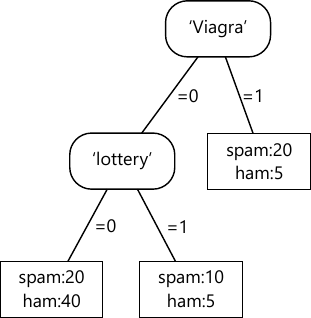MORE IN Machine Learning
SPPU Information Technology (Semester 7)
Machine Learning
December 2015
Total marks: --
Total time: --
INSTRUCTIONS
(1) Assume appropriate data and state your reasons
(2) Marks are given to the right of every question
(3) Draw neat diagrams wherever necessary

Solve any one question from Q1 and Q2
1 (a) Explain predictive and descriptive tasks.
5 M
1 (b) Prove with an example Accuracy = 1-error rate.
5 M

2 (a) Define class probability estimator. State mathematical model of class probability estimator. Is that a predictive or descriptive task? Justify.
5 M
2 (b) What is majority class decision rule? Using following feature tree, write decision rules for majority class.5 M

Solve any one question from Q3 and Q4
3 (a) What is a slack variable? Discuss margin errors.
5 M
3 (b) Explain ridge regression and lasso.
5 M

4 (a) Consider the following three-class confusion matrix.
Calculate precision and recall per class. Also calculate weighted average precision and recall for the classifier.
 Predicted Actual 15 2 3 7 15 8 2 3 45
5 M
4 (b) Explain the term bias-variance dilemma.
5 M

Solve any one question from Q5 and Q6
5 (a) Explain with the help of diagrams and equations Minkowski, Euclidean, Manhattan and Hamming distances.
8 M
5 (b) What is a feature tree? Write the Grow Tree algorithm to generate feature tree. Explain the role of best split in this algorithm.
10 M

6 (a) Explain support and confidence with the help of formulae. Calculate support, and confidence for the following example.
 Transaction Items 1 nappies 2 beer, crisps 3 apples, nappies 4 beer, crisps, nappies 5 apples 6 apples, beer, crisps, nappies 7 apples, crisps 8 crisps
8 M
6 (b) Write an algorithm for K-means clustering. Describe its working in brief using example.
10 M

Solve any one question from Q7 and Q8
7 (a) Distinguish between discriminative learning models and generative learning model with suitable examples.
8 M
7 (b) Define:
1) Bernoulli's distribution.
2) Binomial distribution.
3) MAP decision rule.
4) Maximum likelihood function.
8 M

8 (a) Write a note on Naive Bayes Classification algorithm.
8 M
8 (b) Explain in brief logistic regression. Compare simple regression and logistic regression.
8 M

Solve any one question from Q9 and Q10
9 (a) Explain reinforcement learning.
8 M
9 (b) Explain bagging and boosting as ensemble methods.
8 M

10 (a) Explain data stream and online learning.
8 M